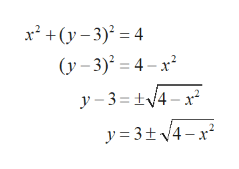# determine if the relation defines y as a function of x.x^2 + (y− 3)^2 = 4

Question
10 views

determine if the relation defines y as a function of x.

x^2 + (y− 3)^2 = 4

check_circle

Step 1

A relation is a function if for every x there is exactly one value of y.

Step 2

Given:  x^2 + (y− 3)^2 = 4.

Solve the given equation in terms of y.help_outlineImage Transcriptionclosex² +(y– 3)² = 4 (у -3)* — 4- х? y – 3 = tV4 – x /4- х у %3 3+v4-х? y = fullscreen
Step 3

The above equation gives two different values of y for each x...

### Want to see the full answer?

See Solution

#### Want to see this answer and more?

Solutions are written by subject experts who are available 24/7. Questions are typically answered within 1 hour.*

See Solution
*Response times may vary by subject and question.
Tagged in

### Other